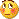ZigZag Channels! - page 581

I don't want to be importunate, but it would be very nice if someone can make this trend lines to update automatically. When I run backtest with this indicator there is only zigzag line updating, but trend lines stays at their initial positions. Is there any way to fix it? I've tried to do this by myself but nothing worked. Sorry for bad english and thanks in advance.495

Could someone post these indicators

Please post these indicators

stljeffbb:
Here is the code for these three indicators written in metastock/tradestation language:

ZIG ZAG VALIDATION

This indicator validates the LAST LEG of Zigzag.

Values returned:

1 = valid leg (not revisable), 0 = invalid leg (revisable).

Do NOT use this indicator in systems or for backtesting.}

perc:=Input("Percent",0.0001,1000,10);

Z:=Zig(CLOSE,perc,%);

last:=ValueWhen(1,( Z > Ref(Z,-1) AND Ref(Z,-1) < Ref(Z,-2) )

OR

( Z Ref(Z,-2) ),

Ref(Z,-1));

pc:=(CLOSE-last) * 100 / last;

pc:= Abs(pc);

SD:=(z>Ref(z,-1) AND Ref(z,-1)>Ref(z,-2)) OR (z<Ref(z,-1) AND Ref(z,-

1)<Ref(z,-2));

res:=If(pc>=perc ,1,0);

ZIG ZAG TREND

{This is a safe indicator as it deals with valid trends only.

You can trade it directly or incorporate it in any other

tool or system.}

Values returned:

1 = Valid uptrend, -1 = valid downtrend }

vr:=Input("Field (0=Ind/tor, 1=Open, 2=High, 3=Low, 4=Close)",0,4,0);

amnt:=Input("Reversal amount",0.0001,1000,10);

md:=Input("Method (1=Percent, 2=Points)",1,2,1);

vr:=If(vr=1,OPEN,If(vr=2,HIGH,If(vr=3,LOW,If(vr=4, CLOSE,P))));

zz0:=If(md=1, Zig(vr,amnt,%), Zig(vr,amnt,\$));

zz1:=Ref(zz0,-1);

zz2:=Ref(zz0,-2);

tr:=ValueWhen(1,zz0>zz1 AND zz1<zz2, zz1);

pk:=ValueWhen(1,zz0zz2, zz1);

PU:=If(md=1,tr+Abs(tr)*amnt/100,tr+amnt);

PD:=If(md=1,pk-Abs(pk)*amnt/100,pk-amnt);

res:=If(vr>=PU AND zz0>zz1,1,

If(vr<=PD AND zz0<zz1,-1,0));

res:=If(res0,res,ValueWhen(1,res0,res));

res

ZIG ZAG THRESHOLD

{This indicator returns the price at which Zigzag Trend (ZZT) will change direction

(the price at which we will have a new confirmed trend)}

vr:=Input("Field (0=Ind/tor, 1=Open, 2=High, 3=Low, 4=Close)",0,4,0);

amnt:=Input("Reversal amount",0.0001,1000,10);

md:=Input("Method (1=Percent, 2=Points)",1,2,1);

vr:=If(vr=1,OPEN,If(vr=2,HIGH,If(vr=3,LOW,If(vr=4, CLOSE,P))));

zz0:=If(md=1, Zig(vr,amnt,%), Zig(vr,amnt,\$));

zz1:=Ref(zz0,-1);

zz2:=Ref(zz0,-2);

tr:=ValueWhen(1,zz0>zz1 AND zz1<zz2, zz1);

pk:=ValueWhen(1,zz0zz2, zz1);

PU:=If(md=1,tr+Abs(tr)*amnt/100,tr+amnt);

PD:=If(md=1,pk-Abs(pk)*amnt/100,pk-amnt);

res:=If(vr>=PU AND zz0>zz1,1,

If(vr<=PD AND zz0<zz1,-1,0));

res:=If(res0,res,ValueWhen(1,res0,res));

top:=HighestSince(1,res>Ref(res,-1),vr);

bot:=LowestSince(1,res<Ref(res,-1),vr);

threshold:=If(res=-1,

If(md=1,bot+Abs(bot)*amnt/100,bot+amnt),If(md=1,top-Abs(top)*amnt/100,top-amnt));

threshold;

Thanks!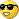20410

What about the exit?Any ideas....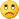Files:
jpy_zgzag.jpg 86 kb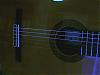31

Zigzag

Is it possible to find ZZ indi with adjustable minimum % of price range?

Thanks

Viat1243612

Great job XArd777!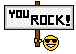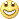The Quadrant lines indeed further in helping trade using zigzag channel, where the price find the SR.

If you can add an alert if price breaking the channel lines it will be great.

Thanks. You da man!matfx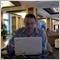Moderator
112855

viat:
Is it possible to find ZZ indi with adjustable minimum % of price range?

Thanks

Viat

Check this thread https://www.mql5.com/en/forum/172952 (may be you will find something there).5921

codersguru:
Hi there!

A new indicator needs your comments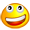!

ZigZag Channels

Coders,

could be this indicator translated to the new zig zag code.

Thanks mate.57

Codeguru, thanks for your kind efforts!

Actually what is the function of this channel? Sorry for my silly question!